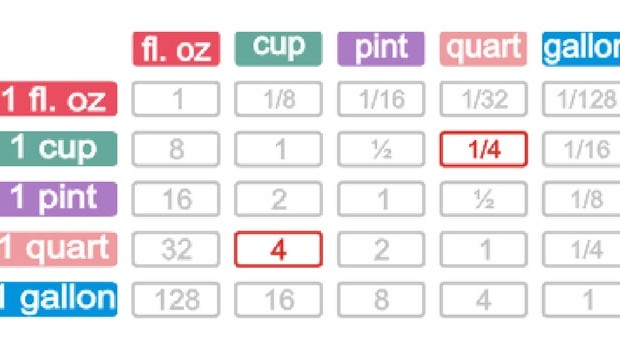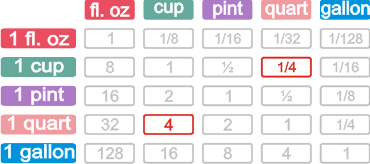How Many Cups In a Quart? Now You KnowHow many cups in a quart? This guide will let you know, easy peasy. Before we answer the burning question of the day, what is a quart anyway? Well, by the US volume unit, a Quart is an imperial unit of measurement, the commonly known symbol for a Quart is simply ” Qt “. As you already figured out, this measuring unit is very popular in the US, but in other parts of the world there are different variations of a Quart, there for the question of how many cups in a quart is a very popular one. the short answer is that 1 Quart is the same or very similar amount of 1 liter.

Below is a very useful conversion chart of measuring how many cups in a Quart:

 Quart Cups 1 4 2 8 3 12 4 16 5 20 6 24 7 28 8 32 9 36 10 40

Why is there such a confusion with the simple question of how many cups in a Quart?

There is much confusion about this question because the US measurement of a Quart is not the same as in Europe, and same goes for other places in the world. So many people are trying to figure out the answer to this depending on where they are in the world. For example, in the UK a Quart is equal to 4.5 cups, and not just 4 as in the US. So it’s easy to see why so many people are interested in this ” simple question ”

If you also want to know about the measuring unit of a cup, the basic thing to know is that a cup is 250ml of liquid. so 4 cups are equal to 1 liter, and as we already know, 1 liter is equal to a Quart